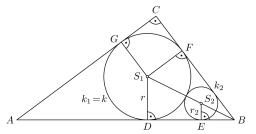# Piece of a wire

Piece of wire is bent into the shape of a triangle. Two sides have length 24 inches and 21 inches.
The angle between these two sides is 55°. What is the length of the third side to the nearest hundredth of an inch?

A: The length of third side is approximately ____ inches.

c =  20.9484 in

### Step-by-step explanation:

Try calculation via our triangle calculator.Did you find an error or inaccuracy? Feel free to write us. Thank you!

Tips for related online calculators
The Pythagorean theorem is the base for the right triangle calculator.
Do you want to convert time units like minutes to seconds?
Cosine rule uses trigonometric SAS triangle calculator.